# Side Effects in FP

It is very important how to handle side effects in functional programming because on the one hand they are necessary to have any interaction with surrounding world and on the other hand if we approach them not corretly we will lose all advantages of Functional Programming.

To better understand role of side effects in functional programming and gain better intuition around "impurity" - it is good to look at this concept from a total function and partial function perspective.

A total function is a function which is defined for every possible input. And of course a partial function is a function which is not defined for some inputs.

An abstract example may be divide a b which is not defined for b==0 but we may find also many more "real life examples"

• A function which process a Text is defined for a all input texts
• but a function which process Text from a file is not defined for not existing files
• A function which applies discount to final price is defined for all discounts and prices
• but a function which applies discount received from external service is not defined for connections which results in timeout.
• A function which display User information are defined for all existing users
• but of course they are not defined for not existing users

Functional Programming is able to handle those situations and convert partial functions into total functions by explicitly definig types for mentioned situations. Those types represents effects (or side effects).

The purpose of this workshop is to gain better understanding and intuition around those mechanisms.

# Part 1 - Happy Paths in Perfect World

As a warm up we will start with some exercises where we don't need to care about any side effects.

#### Tuples and Streams

As a part of our domain we have a purchase which consist of purchase-lines telling us how many units of a given product was purchased.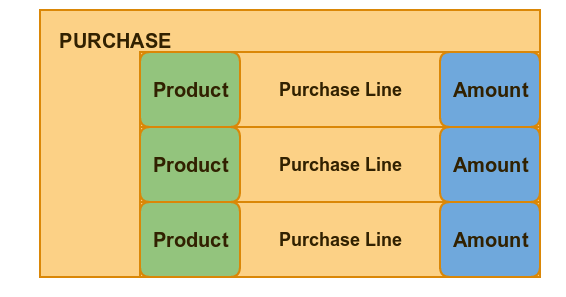In demonstration part we will be presented a new construct Tuple from Javaslang library. A tuple consist of two or more values which types may be different and we have a set of very handlful functions :

• map1 - creates a new tuple with mapped first field
• map2 - the same but for second field
• map BiFunction - maps full tuple with one two-arg function
• map Function Function - maps full tuple with two separate functions
• transform - uses all fields to produce some new type.

We will also use Java8 stream to aply batch operations on all purchase lines.

This will transform a purchase line into a Tuple (product name, amount)

`````` data().purchase1.getLines().stream()
.map(line -> Tuple.of(line.product.name,line.amount))
.forEach(System.out::println);
``````

And the following one is calculating totla number of purchased products :

``````Integer numberOfProductsBought=
data().purchase1.getLines().stream().map(line -> line.amount).reduce(0,Integer::sum);
``````

#### Generating Front End

As Part of our exercise we are going to work with fuinctionality of generating fron html. This exercise should show how to use functional approach to solve familiar problems.

Our Purchase has an optional consultant which has three optional fields

• email
• phone
• picture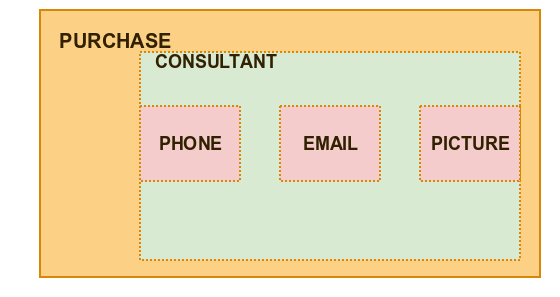The following function converts Consultant to HTML

``````static Function<Consultant,HTML> consultantContactInfo = c -> html(
"<div> Contact your consultant : "+c.name+" "+c.picture.asciiArt+"  <br/>" +
"by email : "+c.email+" or by phone "+c.phoneNumber+"</div>"
);
``````

However we have some unanswered questions - what if consultant is missing? what if there is consultant but some of his fields are missing?

#### Calculating discount

We also have a "business logic" module where we are defining algorithm for applying discount to purchase price. We have there a discount for specific users and also special discount for specific cities.

``````static Function<Set<Customer>,Function<Customer, BigDecimal>> discountsConfiguration = customersWithDiscount->
customer -> customersWithDiscount.contains(customer.name)? new BigDecimal("0.3") : BigDecimal.ZERO;

static Function<Customer, BigDecimal> discountForCustomer=discountsConfiguration.apply(new HashSet(Arrays.asList("Joe")));

static Function<String, BigDecimal> discountForACity = city -> city.equals("Lodz") ? new BigDecimal("0.2") : BigDecimal.ZERO;
``````

### Exercises

Exercise Level 1: Tuples

In this exercise you have to apply map1,map and transform to properly transform data inside a tuple.

Exercise Level 2: Streams

Here your are going to implement some simple operations on streams

• Sum all elements
• Parse and then sum all elements
• Join string elements

Exercise Level 3: Generating HTML

• Implement high order fucntion which wraps given html into div block
``````static <A> Function<A,HTML> insideDiv(Function<A,HTML> toHtml){
return null;
}
``````
• You have prepared function which generates customer String information. Use it to create final version of customer to html conversion :
`````` static Function<Customer,HTML> cystomerContactInfo = c -> html(
"Customer contact : "+c.name+" with email : "+c.email+""
);

//EXERCISE3 - decorate customerContactInfo so it is wrapped in div block
static Function<Customer,HTML> customerToHtml= ???;
``````
• With prepared conversion of product to string - implement purchase line to html conversion - use assertions defined in exerciseLevel3 method
``````static Function<Product,String> productToString= p -> p.name +" : "+p.price+"\$";

//EXERCISE3 - use productToString to build purchase line representation
// check Exercise 3 for assertions - "<div>tv : 300\$ : 2</div>"
static Function<PurchaseLine,HTML> purchaseLineToHtml= insideDiv(
???
);
``````

Question : how you handled all data variances? What if some information is missing. How would you modify the code. How your modification would influence testing

Exercise Level 4: Calculating discount

With provided mechanism granting discount to specific user and cities

``````static Function<Set<Customer>,Function<Customer, BigDecimal>> discountsConfiguration = customersWithDiscount->
customer -> customersWithDiscount.contains(customer.name)? new BigDecimal("0.3") : BigDecimal.ZERO;

static Function<Customer, BigDecimal> discountForCustomer=discountsConfiguration.apply(new HashSet(Arrays.asList("Joe")));

static Function<String, BigDecimal> discountForACity = city -> city.equals("Lodz") ? new BigDecimal("0.2") : BigDecimal.ZERO;
``````

Write three variants of method counting discount according to algorithm :

1. if there is discount for given customer - then return it
2. if there is not check if there is discount for city
3. if there is none of them then return zero

4. second version return provided default value instead of zero

5. third version call provided supplier to retrieve default value
`````` static Function<Customer, BigDecimal> discountV1 = ???;
static Function<BigDecimal,Function<Customer, BigDecimal>> discountV2 = ???;
static Function<Supplier<BigDecimal>,Function<Customer, BigDecimal>> discountV3 = ???;
``````

# Part 2 - Optionality Effect

First effect which we are going to learn is perhaps the easiest one to grasp - an optionality effect

When to use it : when there is a possibility of missing a value normally in Java null is used but null generates two main problems.

1. Actually you don't know if given reference may be null by contract or not (is this null "business null" or "error null"?)
2. If you have null you actually don't know "what is the type of this null because nul, doesn't have type..."

Solution to this problem is to "Materialize" optionality effect into Type System.

#### Java8 Optional

In the standard library we can use Optional Type added in Java8. It has couple of basics operators so it is possible to apply pure data transformation to its context.``````print(" * MAPPING IN OPTIONAL CONTEXT");
Function<String,Integer> parse = Integer::parseInt;
Function<Integer,Double> addTax= price -> price + (0.23*price);

Optional<String> potentialStringValue = Optional.of("10");
print("  * Gross 10 from optional: "+ potentialStringValue.map(readGrossPrice));
``````

At the end we have couple way of extracting value from Optional

``````potentialStringValue.map(readGrossPrice).orElse(0.0);
Supplier<Double> longCalculations=()->0.0;
Double grossOrDefault = potentialStringValue.map(readGrossPrice).orElseGet(longCalculations);
``````

#### JavaSlang Option

Java8 Optional has only a few methods and is missing some very useful combinators. One of those combinators is orElse(OtherOption)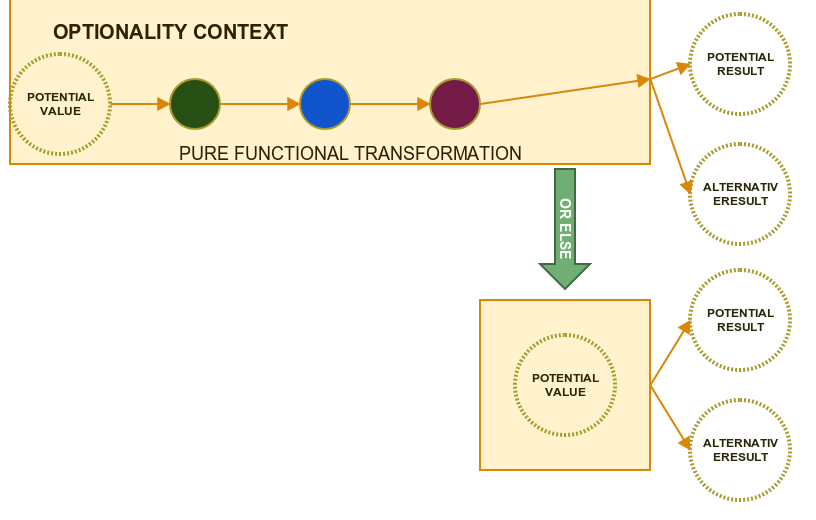Fortunatelly we can use an external library Javaslang which provides many useful (and missing in standard library) abstractions.

First of all it is very easy to convert from Optional to Option and the other way around

`````` Option<String> slangSome=Option.of("10");

print("  * TO JAVA OPTIONAL : "+slangSome.toJavaOptional());
print("  * FROM JAVA OPTIONAL : "+Option.ofOptional(potentialStringValue));
``````

Then we have access to mentioned orElse operator.

``````ption.ofOptional(potentialStringValue)
.orElse(Option.of("5"))
.forEach(gross->print("  * PARSED OPTION SOME "+gross));
``````

### Exercises

Exercise Level 1: Creating Optional & Option

In this section you are going to practice creating optionals and option in couple simple exercises.

Exercise Level 2: Transforming optional data

First you need to implement methodwhich safely retrieve data from map (returns Empty optional instead of null)

``````static <A,B> Optional<B> findInMap(Map<A,B> map,A key){
return null;
}
``````

In second Part you need to use second source/map & orElse to find price data and calcualte tax. Notice how happy path function is applied in transformation chain.

Exercise Level 3: Display data with optional field

Product type has an optional field description use this field to generate Product HTML representation

``````static HTML displayProduct(Product product){
return ???;
}
``````

Exercise Level 4: Discount Calculation

Similarly to previous section you have some functions which calcualtes discount for Users and Cities - however this time those functions return Javaslang Option

``````Function<Set<Customer>,Function<Customer, Option<BigDecimal>>> discountsConfiguration = customersWithDiscount->
customer -> customersWithDiscount.contains(customer.name)? Option.of(new BigDecimal("0.3")) : Option.none();

Function<Customer, Option<BigDecimal>> discountForCustomer=discountsConfiguration.apply(new HashSet(Arrays.asList("Joe")));

Function<String, Option<BigDecimal>> discountForACity = city ->
city.equals("Lodz") ? Option.of(new BigDecimal("0.2")) : Option.none();
``````

Rules are the same :

1. if there is discount for given customer - then return it
2. if there is not check if there is discount for city
3. if there is none of them then return zero
``````Function<Customer,BigDecimal> calculateDiscount=???;
``````

# Multiple Optionals

In previous section we worked with optional data which was isolated. But what if one optional data needs to interact with another optional data?

Imagine that we want to lookup a Customer in some database in two steps :

1. First step : parse Input Number passed in String format.
2. Second step : use parsed id to lookup for optional user
``````Function<String, Optional<Integer>> parse = s -> {
try {
return Optional.of(Integer.parseInt(s));
} catch (Exception e) {
return Optional.empty();
}
};

Function<Integer, Optional<String>> lookup = id -> (id == 5) ? Optional.of("Zygfryd") : Optional.empty();
``````

The problem with using map to apply second function is that second function returns an effect by itself and we would receive doubled effect as a result

``````Optional<Optional<String>> result1 = parse.apply("10").map(lookup);
``````

The solution is relatively simple - each time when you combine function which also return an effect then use flatMap instead of map.

``````Optional<String> flatMapResult = parse.apply("10").flatMap(lookup);
``````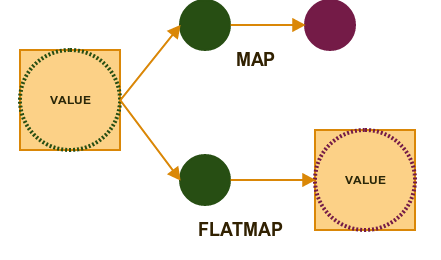#### Javaslang "FOR"

Javaslang library gives us another interesting way of combining effects. It is inspired by scala's for-comprehension

``````Iterator<Integer> javaslangResult = For(
Option.of(1), Option.of(2), Option.of(3)
).yield((a, b, c) -> a + b + c);
``````

So first we are passing all effects and then - in yield section - we are passing function which takes as a parameters all values which may be represented by effects.

### Exercises

Exercise Level 1: Combining optional information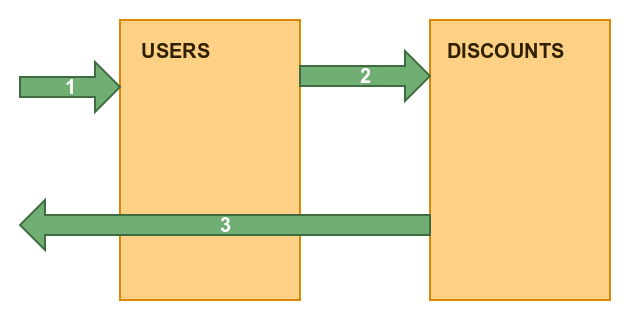You have two maps representing data stores :

``````Map<String, Integer> searchId = new HashMap<String, Integer>() {{
put("Joe", 1);
put("Jane", 2);
}};
Map<Integer, BigDecimal> discountForUser = new HashMap<Integer, BigDecimal>() {{
put(1, new BigDecimal("0.3"));
}};
``````

You need to find an optional id in the first datastore and then use it to find an optional discount in the second datastore. And finally use this discount to calculate final price.

Exercise Level 2: Displaying hierarchy of optional fields

You need to display consultant info from purchase but ... :

• Purchase may not have a consultant
• Consultant may have an email
• But instead of an email he/she may have a phone number

Exercise Level 3: Displaying hierarchy of optional fields with FOR

Use Javaslang For to display consultant info. This time you will have to use different notation which allow you to make one effect dependant on another

``````Function<Purchase, String> consultantInfo = p -> API.For(Option.of(p.consultant), (Consultant c) -> ???
``````

# Error Effect

The limitation of an Option appears when we are not only interesting that something is missing but we want to know what exactly is missing and why it is missing. This is the moment when an Error effect appears.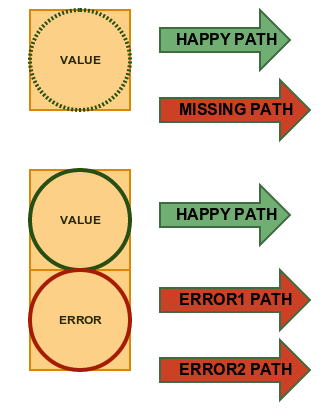Standard Java library doesn't have proper error representation so we are going to use Try type from the Javaslang library.

``````Function<String, Try<Integer>> tryParse = s -> Try.of(() -> Integer.parseInt(s)); //LAZY !! EXPLAIN
BiFunction<Integer, Integer, Try<Integer>> tryDivide = (a, b) -> Try.of(() -> a / b);
``````

Notice that computation which potentially triggers error are passed lazily to Try - we will shortly explain during workshops why and what are the consequences.

#### extract & recover

Try provides set of very useful operators to extract value whether computation ended with a success or an error

``````tryParseAndDivide.apply("4", "2").onSuccess(result -> print("  * success : " + result));

(...)
.getOrElseGet(exception -> (exception instanceof ArithmeticException) ?
html("<error>undefined operation </error>") :
html("<error>wrong input data </error>")
);
``````

### Exercises

Exercise Level 1: Combining Error Effects

You have three Try effects

``````Try<Integer> try1 = Try.success(1);
Try<Integer> try2 = Try.success(2); //Check what will happen when this one is failure
Try<Integer> try3 = Try.success(3);
``````

Calculate sum of their values :

• with flatMap and map
• with FOR

Exercise Level 2: Connecting to purchase datastore

You can receive purchase data through two connections :

``````Function<Integer, Try<Purchase>> readPurchase = id -> Try.failure(new ConnectException("Timeout"));
Function<Integer, Try<Purchase>> newConnection = id ->
id == 1 ? Try.success(data().purchase1) : Try.failure(new ConnectException("Timeout"));
``````

The first one always fail and the second one works only for some ids. Use Try to connect first with "readPurchase" and in case of an error with "new connections". Then use provided code template to properly handle computation flow

Exercise Level 3: Notify about error

We have pure function which substract discount from a final price.

``````//Happy Path Without Effects
Function<BigDecimal, Function<BigDecimal, BigDecimal>> happyPath = price -> discount ->
price.subtract(price.multiply(discount));
``````

We also have a state which simulates outside world

``````//State to store notifications

//Stateful function which adds notification to state
``````

The problem is that read discount function may return an error

``````Function<Customer, Try<BigDecimal>> readDiscount = c ->
c.name.equals("Joe") ? Try.success(new BigDecimal("0.3")) :
Try.failure(new IllegalAccessException("ILLEGAL ACCESS FOR " + c.name));
``````

In the exercise you need to read discount , send notification in case of an error and then convert Try to Option

``````//1) read discount
//2) apply happy path for price BigDecimal(100)
//3) if failure - notify
//4) convert to an option
Function<Customer, Option<BigDecimal>> applyDiscount = ???
``````

# Time Effect

This is a very interesting effect because in this exercise we can directly observe physical infuence of time. In the real world computation are not always instant but many times we need to wait for a response from external services and from time to time - we may need to wait forever...The main abstraction here is CompletableFuture from Java8

``````CompletableFuture.supplyAsync(() -> readPurchase.apply(500));
``````

In the exercises we are also see how to combine it with an Error effect.

``````Try<String> happyPathApplied = Try.of(() -> readingPurchase.thenApply(happyPath).get(1, TimeUnit.SECONDS));
``````

### Exercises

Exercise Level 1: Combining Futures

You have two services which respond after some time:

``````Supplier<CompletableFuture<BigDecimal>> discountOfTheDay = () ->
CompletableFuture.supplyAsync(
() -> {
sleep(500);
return new BigDecimal("0.1");
}
);

Function<Customer, CompletableFuture<BigDecimal>> discountForUser = c -> CompletableFuture.supplyAsync(
() -> {
sleep(600);
return c.name.equals("Joe") ? new BigDecimal("0.3") : BigDecimal.ZERO;
}
);
``````

And then use : "thenCombine(BIFunction)" to calcualte final value.

Exercise Level 2: Combining Different Effects

We have a function which computes result in some time and also may result in error :

``````Function<String, CompletableFuture<Integer>> parseService = input ->
CompletableFuture.supplyAsync(() -> {
sleep(500);
return Integer.parseInt(input);
}
);
``````

You need to define a function which handles both path - success and error by mapping them to Try type

``````//EXERCISE - Map possible future values into try
BiFunction<Integer, Throwable, Try<Integer>> handleFuture = ???;

CompletableFuture<Try<Integer>> parseCorrect = parseService.apply("1").handle(handleFuture);
``````

Then observe how to safely extract value when there can be a timeout exception :

``````Try<Integer> incorrectResult = Try.of(() -> parseIncorrect.get(1, TimeUnit.SECONDS)).getOrElseGet(Try::failure);
``````

Exercise Level 3: Join Combinator

In the previous exercise we saw that sometimes you may end with a Type

``````Try<Try<A>>
``````

We can create universal function which can join this type to receive single

``````
implement this funciton

```Java
static <A> Try<A> join(Try<Try<A>> input) {
return ???;
}
``````

And oberve how it cna be used in computation

``````Try<Try<Integer>> parsingResultWrongInput = Try.of(() -> parse.apply("aaa").get(1, TimeUnit.SECONDS));
Try<Integer> joinWrongInput = join(parsingResultWrongInput);
``````

# Bonus

• As a bonus (if there is time) we may discuss why Java8 Optional is missing many useful combinators and why it is not implementing Serializable

• How when using null we are losing all information about missing type and what are consequences